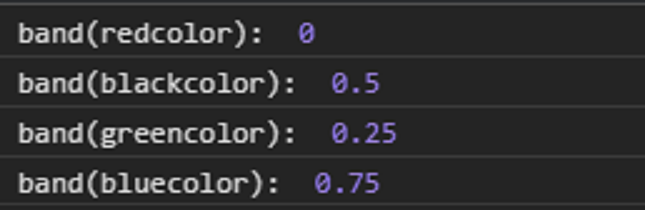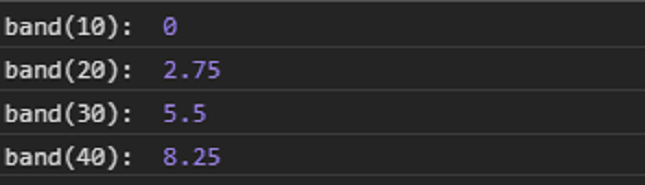Related Articles

# D3.js band.domain() Function

• Last Updated : 23 Sep, 2020

The band.domain() function in d3.js is used to set the domain of the scale. The first value that is present in the domain will be mapped to the first band in the range array and so on.

Syntax:

`band.domain([domain]);`

Parameters: This function accepts single parameters as given above and described below.

• domain: This parameter sets the domain of the scale i.e the minimum and the maximum value.

Return Values: This function does not return anything.

Example 1:

## HTML

 ` ``<``html` `lang` `= ``"en"``> ``<``head``> ``    ``<``meta` `charset` `= ``"UTF-8"` `/> ``    ``<``meta` `name` `= ``"viewport"``        ``path1tent = "``width``=``device``-width, ``        ``initial-scale` `= ``1``.0"/> ``     ``<``script` `src``=``        ``"https://d3js.org/d3.v4.min.js"``>``    ``    `` `` ` `<``body``> ``    ``<``script``> ``      ``// Create band scale with domain``      ``// and range``      ``var band = d3.scaleBand()`` ` `      ``// Setting domain for the scale``      ``.domain(["redcolor", "greencolor",``             ``"blackcolor", "bluecolor"]);`` ` `      ``console.log("band(redcolor): ",``              ``band("redcolor"));``      ``console.log("band(blackcolor): ",``              ``band("blackcolor"));``      ``console.log("band(greencolor): ",``              ``band("greencolor"));``      ``console.log("band(bluecolor): ", ``              ``band("bluecolor"));                        ``    `` `` ```

Output:Example 2:

## HTML

 ` ``<``html` `lang` `= ``"en"``> ``<``head``> ``    ``<``meta` `charset` `= ``"UTF-8"` `/> ``    ``<``meta` `name` `= ``"viewport"``        ``path1tent = "``width``=``device``-width, ``        ``initial-scale` `= ``1``.0"/> ``   ` `    ``<``script` `src` `=``      ``"https://d3js.org/d3.v4.min.js"``>``    ```` `` ` `<``body``> ``    ``<``script``> ``        ``// Creating the band scale with``        ``// specified domain and range.``        ``var band = d3.scaleBand()`` ` `        ``// Setting domain for the scale``                 ``.domain([10, 20, 30, 40])``                 ``.range([0, 11]);`` ` `        ``console.log("band(10): ", band(10));``        ``console.log("band(20): ", band(20));``        ``console.log("band(30): ", band(30));``        ``console.log("band(40): ", band(40));``    `` `` ```

Output:Hey geek! The constant emerging technologies in the world of web development always keeps the excitement for this subject through the roof. But before you tackle the big projects, we suggest you start by learning the basics. Kickstart your web development journey by learning JS concepts with our JavaScript Course. Now at it’s lowest price ever!

My Personal Notes arrow_drop_up